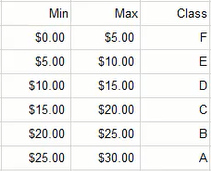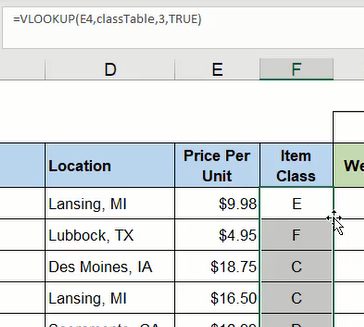The Lookup, Index, and Match functions include some advanced techniques that will be explored in this chapter. We'll begin with a spreadsheet containing data of ingredients and consumption history for eight weeks from a student-run business. The data includes prices, vendors, and locations. We need to fill in the item class information determined by price ranges.

Use this workbook for the chapter.

We will create a table including Min (minimum), Max (maximum), and Class columns. Each row will determine a separate price range beginning with $0.00 minimum to$5.00 maximum and increasing by $5.00 thereafter. The final range is$25.00 to $30.00 which will be designated class A with lower ranges descending through B, C, D, E, and F. The table should be renamed for quick reference; for this example, it is named classTable. (See Figure 27.2)Figure 27.2 We'll return to the item class cells in the data spreadsheet and use the VLOOKUP function to look up the Price Per Unit within the designated classTable array to identify the class letter in column 3. Lastly, the function needs to return a TRUE value for an approximate match. Note: A TRUE argument determines a value based on approximation within a range, whereas a FALSE argument can only return a value if the target reference matches the minimum or maximum range numbers. In this exercise, the target references vary between the specific range points, so the function needs to find an approximate value.Figure 27.3 ## Row and Column Functions The Row and Column functions ask for an optional reference argument. The functions work by identifying the referenced cell's numerical value in the spreadsheet. For example, =ROW(A1) will return a value of 1 because the referenced cell, A1, is in row 1 of the spreadsheet. The function =ROW(E1) will also return a value of 1 because the referenced cell is still in row 1. If the reference cell is in a different row of the spreadsheet, the function will return the relevant value of the row. The Column function works similarly to identify the numerical position of the column regardless of the referenced cell's row position. If no cell is referenced in the Row or Column function, the value of the function's current cell position will be returned. For example, if =COLUMN() is inserted in cell E10, it will return the value 5 because the function's cell is in column E which is the fifth column in the spreadsheet. On their own, the Row and Column functions are not particularly useful. However, when they are used in conjunction with another function, they can simplify that function. ## Combining VLOOKUP and Column In this exercise, we want to create a dashboard for Figure 27.1's data to look up information about a particular ingredient. We can copy and paste the data spreadsheet's column labels to a new spreadsheet and insert a data validation list of the ingredients under the appropriate Ingredient column. Next, we need to write a VLOOKUP function beginning with the Vendor column. 1. Type =VLOOKUP and a left parenthesis ( ( ) to begin the function. 2. Select and anchor the cell containing the data validation list of ingredients to identify the lookup value and type a comma ( , ). 3. Select the data table array wherein the lookup will be performed and type a comma ( , ). 1. You may consider naming the table for quick reference. In Figure 27.4, the table array was named table. 4. Type COLUMN and a left parenthesis ( ( ). 5. Select a cell in the B column followed by a right parenthesis ( ) ) to close the function. 1. In this step, we want to reference column B because the Vendor data is in the second column of the data spreadsheet. By using the Column function, it acts as a placeholder to return the value 2 to indicate the lookup will look in the second column of the data table. Furthermore, copying this completed function forward will adjust the Column function to reference subsequent column values; it will prevent us from manually modifying the value each time. 6. Type FALSE to specify an exact match. 7. Type a right parenthesis ( ) ) and press Enter to complete the function (shown in Figure 27.4 as =VLOOKUP($C$10,table,COLUMN(Dashboard!B$1),FALSE)).
8. Reselect the completed cell.
9. Press the small square in the bottom-right corner and drag across the remaining dashboard columns to copy the function.

Note: Formatting may need to be reapplied for the data to match with the data spreadsheet.

The completed dashboard should now reflect the data on the data spreadsheet for the selected ingredient.

## Index and Match

The Index and Match functions can accomplish the same result. In this case, the Match function working within Index is used to search for and match a row in the data table with a designated value. Then it draws a value from a specified column number which will be identified using the Column function again.

1. Type =INDEX and a left parenthesis ( ( ) to begin the function.
2. Select the data table array wherein the lookup will be performed and type a comma ( , ).
1. As suggested in the previous section, you may consider naming the table for quick reference. In this case, the table array was named table.
3. Type MATCH and a left parenthesis ( ( ).
4. Select and anchor the cell containing the data validation list of ingredients to identify the lookup value and type a comma ( , ).
5. Select and anchor the column listing ingredients from the datasheet to identify the data to be searched and matched with the previous step's argument, and then type a comma ( , ).
6. Type 0 (zero) to indicate an exact match, and type a right parenthesis ( ) ) to close the Match function.
7. Type COLUMN and a left parenthesis ( ( ).
8. Select a cell in the B column and type a right parenthesis ( ) ) to close the Column function.
9. Type a second right parenthesis ( ) ) to close the Index function.
10. Press Enter to complete the function (shown as =INDEX(table,MATCH($C$10,Ingredients!$B$4:$B$15,0),COLUMN(B1)) in Figure 27.5).

## XLOOKUP Function

The final function that we can use to lookup information about a particular ingredient from the data spreadsheet is the XLOOKUP function. XLOOKUP works by looking up a designated value from a selected array and returning the associated value of another related array. Additionally, the XLOOKUP function can return a predetermined value or textual note if the initial lookup value cannot be found.

1. Type =XLOOKUP and a left parenthesis ( ( ) to begin the function.
2. Select and anchor the cell containing the data validation list of ingredients to identify the lookup value and type a comma ( , ).
3. Select and anchor the column of ingredients in the data table and type a comma ( , ).
4. Select the column of vendors in the data table and anchor only the row reference, then type a comma ( , ).
5. Type a textual note in quotation marks such as "Not found" to be displayed if a match cannot be found, then type a comma ( , ).
6. Type a 0 (zero) to indicate an exact match.
1. Note: The last optional argument labeled "search mode" is best for a larger dataset wherein indicating an order to begin the search would be beneficial. In this case, the argument will be omitted.
7. Type a right parenthesis ( ) ) and press Enter to complete the function (shown as =XLOOKUP($C$10,Ingredients!$B$3:$B$15,Ingredients!C$4:C$15,"Not found",0) in Figure 27.6).